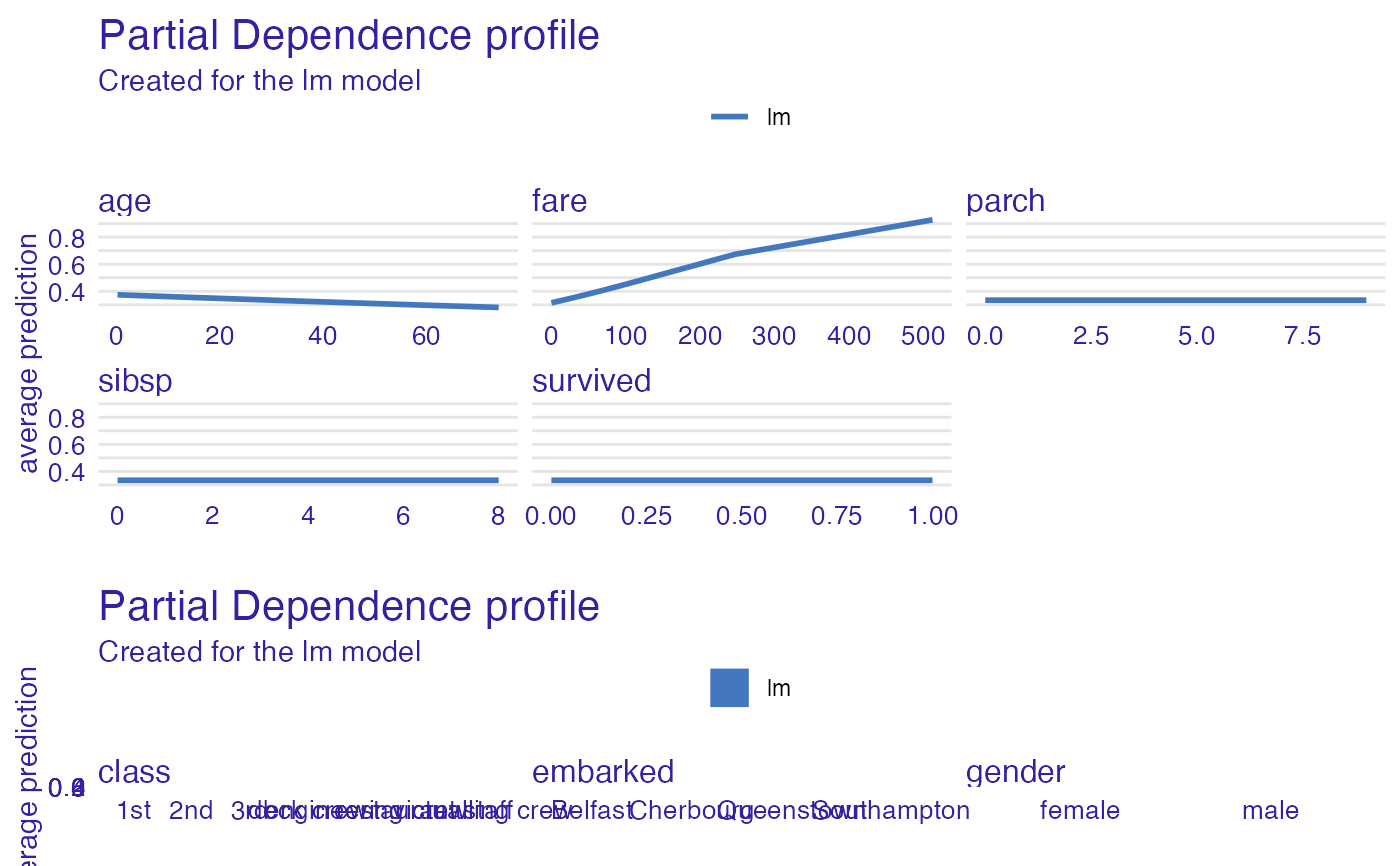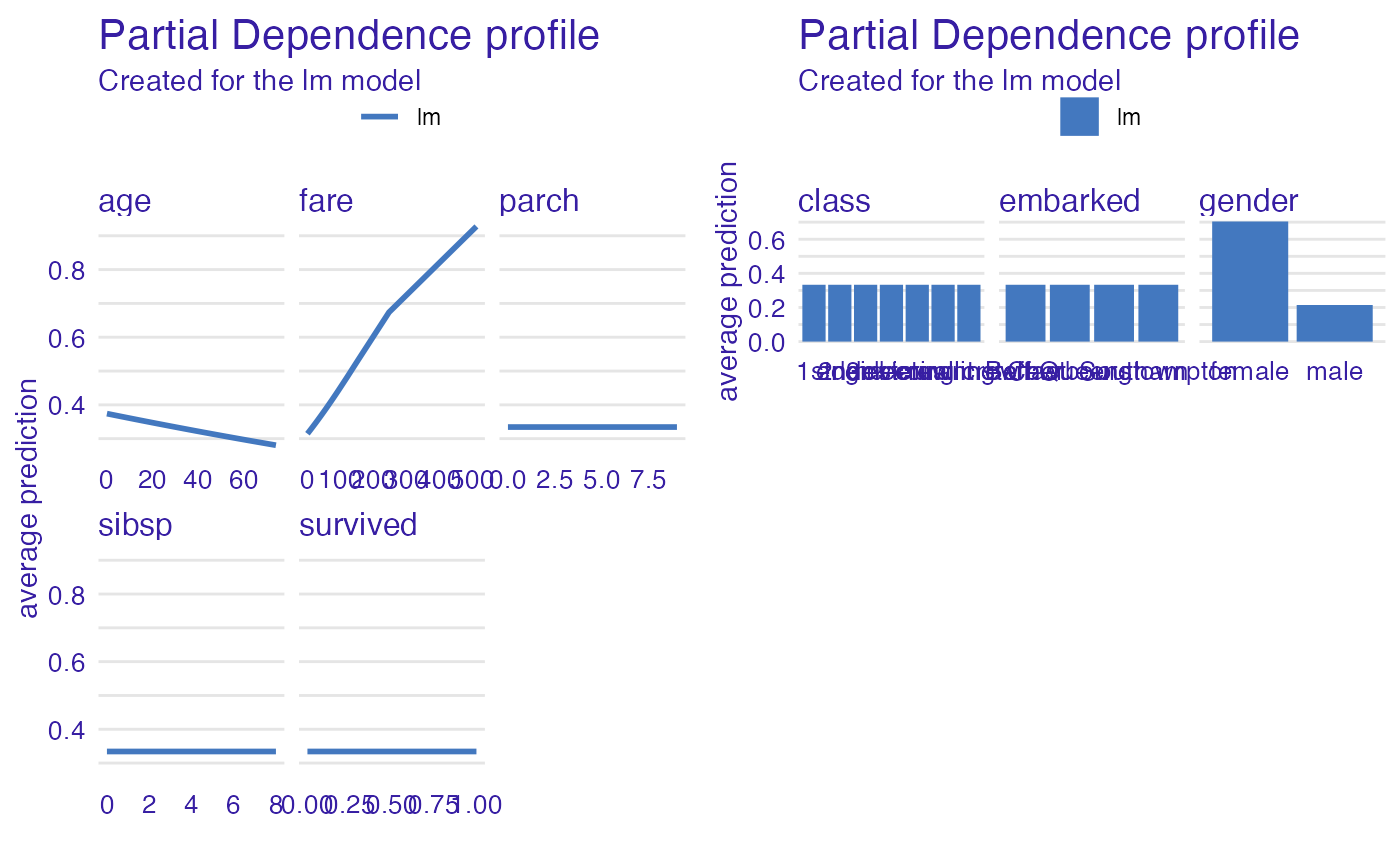This is an aesthetically efficient implementation of the grid.arrange

bind_plots(..., byrow = FALSE)

## Arguments

... (ggplot) ggplot objects to combine. (logical) if FALSE (the default) the plots are bind by columns, otherwise the plots are bind by rows.

## Value

(gtable) A plottable object with plot().

## Author

https://github.com/harell

## Examples

# \donttest{
library("DALEX")
library("ingredients")

titanic_glm <- glm(survived ~ gender + age + fare,
data = titanic_imputed, family = "binomial")

explain_glm <- explain(titanic_glm,
data = titanic_imputed,
y = titanic_imputed\$survived,
verbose = FALSE)

pdp_numerical <- partial_dependence(explain_glm, N = 50, variable_type = "numerical")
pdp_categorical <- partial_dependence(explain_glm, N = 50, variable_type = "categorical")

# Bind plots by rows
bind_plots(plot(pdp_numerical), plot(pdp_categorical), byrow = TRUE)# Bind plots by columns
bind_plots(plot(pdp_numerical), plot(pdp_categorical), byrow = FALSE)# }